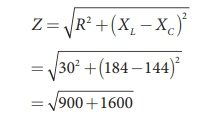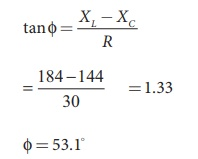Home | | Physics 12th Std | Quality factor or QŌĆōfactor

# Quality factor or QŌĆōfactor

It is defined as the ratio of voltage across L or C to the applied voltage.

Quality factor or QŌĆōfactor

The current in the series RLC circuit becomes maximum at resonance. Due to the increase in current, the voltage across L and C are also increased. This magnification of voltages at series resonance is termed as QŌĆōfactor.

It is defined as the ratio of voltage across L or C to the applied voltage.Q-factor = Voltage across LorC / Applied voltage

At resonance, the circuit is purely resistive. Therefore, the applied voltage is equal to the voltage across R.The physical meaning is that QŌĆōfactor indicates the number of times the voltage across L or C is greater than the applied voltage at resonance.

Since the phase angle is positive, voltage leads current by 53.1 for this inductive circuit.

EXAMPLE 4.22

Find the impedance of a series RLC circuit if the inductive reactance, capacitive reactance and resistance are 184 ╬®, 144 ╬® and 30 ╬® respectively. Also calculate the phase angle between voltage and current.

Solution

XL = 184 ╬®; XC = 144 ╬®

R = 30 ╬®

(i ) The impedance isImpedance, Z = 50 Ōä”

(ii) Phase angle isŽå = 53.1

EXAMPLE 4.23

A 500 ╬╝H inductor, 80/ŽĆ2 pF capacitor and a 628 ╬® resistor are connected to form a series RLC circuit. Calculate the resonant frequency and Q-factor of this circuit at resonance.

Solution

L=500├Ś10-6H; C = 80/ŽĆ2 ├Ś10ŌłÆ12 F; R = 628Ōä”

(i) Resonant frequency isQ =12.5

EXAMPLE 4.24

Find the instantaneous value of alternating voltage Žģ = 10 sin(3 ŽĆ├Ś104 t) volt at i) 0ŌĆås ii) 50 ╬╝s iii) 75 ╬╝s.

Solution

The given equation is Žģ = 10sin (3 ŽĆ├Ś104 t)EXAMPLE 4.25

The current in an inductive circuit is given by 0.3 sin (200t ŌĆō 40┬░) A. Write the equation for the voltage across it if the inductance is 40 mH.

Solution

L = 40 ├Ś 10-3 H; i = 0.1 sin (200t ŌĆō 40┬║)

XL = ŽēL = 200 ├Ś 40 ├Ś 10-3 = 8 Ōä”

Vm = Im XL = 0.3 ├Ś 8 = 2.4 V

In an inductive circuit, the voltage leads the current by 90o Therefore,

v = Vm sin ( Žēt +90┬║)

v = 2 . 4 sin(200t ŌłÆ40 + 90 ┬║)

v = 2 . 4 sin(200t +50 ┬║)volt

Tags : Definition, Explanation, Formulas, Solved Example Problems | Alternating Current (AC) , 12th Physics : Electromagnetic Induction and Alternating Current
Study Material, Lecturing Notes, Assignment, Reference, Wiki description explanation, brief detail
12th Physics : Electromagnetic Induction and Alternating Current : Quality factor or QŌĆōfactor | Definition, Explanation, Formulas, Solved Example Problems | Alternating Current (AC)